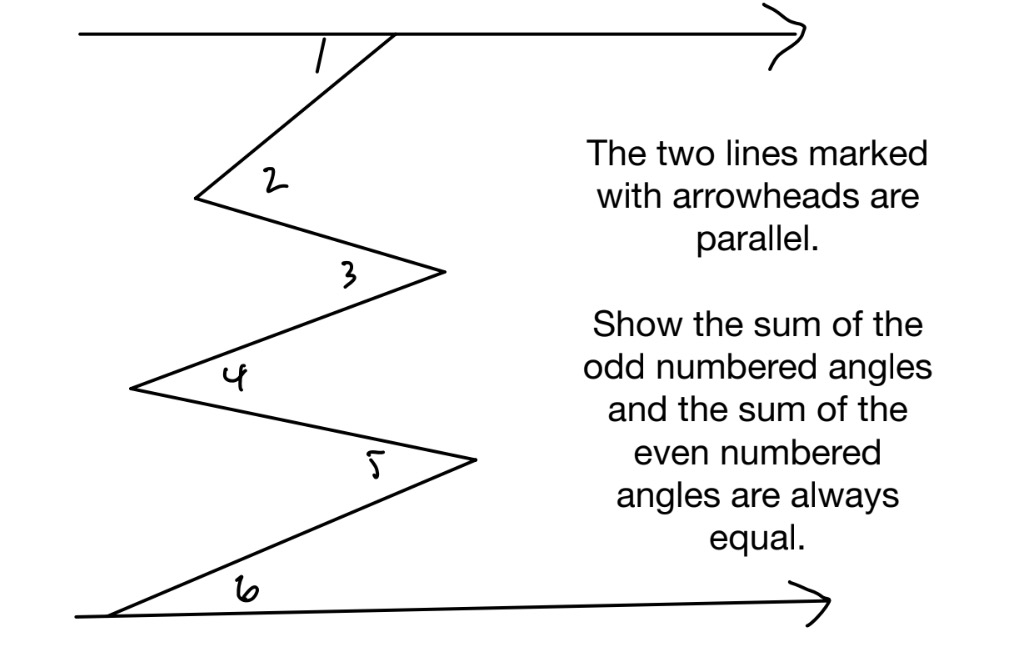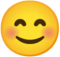## Zig-Zag Angles

Visible to anyone in the world
Edited by Richard Walker, Saturday, 16 Sep 2023, 23:03Share post

### New comment

Hi, Richard.

I think the answer is like this.

Draw the lines through the vertices of angles 2,3,4,5, parallel to the lines marked with arrowheads. If two lines are parallel, alternate angles are equal. So,

(1)angle 1 is equal to upper half of angle 2

(2)lower half of angle 2 is equal to upper half of angle 3

(3)lower half of angle 3 is equal to upper half of angle 4

(4)lower half of angle 4 is equal to upper half of angle 5

(5)lower half of angle 5 is equal to angle 6.

Therefore, the sum of the odd numbered angles and the sum of the even numbered angles are always equal.

### New comment

Yes very good, that's right! Rather a neat little problem I thought